﻿ 基于CVC创业链声誉的微分对策研究

# 基于CVC创业链声誉的微分对策研究Research on Differential Countermeasures Based on CVC Entrepreneurship Reputation

Abstract: As an important member of venture capital investment, company venture capital has been rapidly developed in recent years. It has become an important research topic that how to make the com-pany venture capital can effectively play its role. From the perspective of the reputation of the company venture capital and entrepreneurship chain, this paper uses the method of stochastic differentiation to study the cooperation between the company venture capital and the startups, and obtains the optimal strategy under the Nash game and Stackelberg game that startups dominant. Then the optimal returns under the two games are compared and analyzed. The result shows that under the Nash game condition, the optimal return of CVC is higher than that obtained under Stackelberg game condition, and the optimal return of start-up enterprise is lower compared with the optimal return obtained under Stackelberg game condition.

1. 引言

CVC最先出现在美国，从出现时间上来看，公司创业投资活动起源于传统创业投资之后，两者相差将近20年  。对于CVC的定义，国内外学者均有着不同的看法。Keil认为CVC是大企业进行外部投资的一种形式，并且分析了与公司其他的投资行为(如过渡协议)之间的不同点  。Kann认为CVC是指企业对未上市的创业企业的股权投资行为，属于企业的战略投资，带有明确的商业战略目标  。美国风险投资协会给出的官方定义是：公司创业投资是非金融类的企业从事创业投资项目，或其下属机构所进行的直接投资，这种投资的目的在于寻找与母公司战略、技术等相匹配的创业企业并对其进行投资，在投资过程中能够与之产生协同效应。晏钢将CVC定义为非金融企业在其内部和外部所进行的创业投资活动  。

CVC投资过程是一个漫长的投资过程，连续的时间无疑是CVC创业链成员间合作的重要影响因素，连续时间背景下的博弈充斥着CVC创业链合作的整个过程。连续的时间对CVC创业链上各成员决策的影响也非常复杂，因为CVC投资模式的复杂性，CVC创业链本身也相当复杂。因此，为了能够更好地分析这一问题，本文将CVC创业链简化为由单个CVC与单个创业企业组成的CVC创业链。同时，由于CVC的投资目的的特殊性，CVC创业链上不仅仅考虑财务收益，需要将战略收益纳入考虑范围内。由于CVC创业链的总体战略难以确定以一定的比例在二者之间分配，故只将CVC创业链上所获取的财务收益在二者之间进行分配，故而CVC创业链上的成员之间的博弈就成为一个微分博弈，或者说是微分对策问题。为了解决这一问题，本文首先构建了CVC和创业企业的支付函数，得到了各自的目标函数，Nash博弈和创业企业主导的Stackelberg博弈两种情形下的博弈模型，随后通过逆向归纳法分析模型，并利用算例对两种博弈下的均衡结果进行分析，期望能够为CVC创业链上合作双方的声誉的维护与努力程度提供了依据，为双方的长期稳定发展指明了方向。

2. 模型的构建

2.1. 变量的定义

1) t：时间变量；

2) ${e}_{CVC}\left(t\right)/{e}_{En}\left(t\right)$ ：CVC/创业企业在CVC创业链声誉上的维护与努力水平；

3)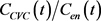：CVC/创业企业的CVC创业链声誉的维护成本；

4)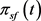：CVC创业链在时刻t的总财务收益；

5) ${\pi }_{CVCs}\left(t\right)/{\pi }_{Ens}\left(t\right)$ ：CVC/创业企业在时刻t的战略收益；

6) ${\prod }_{CVC}\left(t\right)/{\prod }_{En}\left(t\right)$ ：CVC/创业企业在时刻t的收益(包括战略收益与财务收益)；

7)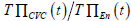：CVC/创业企业在全部合作时间的收益；

8)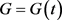：CVC创业链声誉；

9) $\eta$ ：CVC对创业企业的激励因子。

2.2. 基本假设

1) 市场营销领域的研究指出声誉维护成本是努力水平的增函数，且呈现出严格上凸的特点，则设两者之间满足：

${C}_{CVC}\left(t\right)=\frac{{u}_{cvc}\left(t\right)}{2}{e}_{CVC}^{2}\left(t\right)$ (1)

${C}_{En}\left(t\right)=\frac{{u}_{En}\left(t\right)}{2}{e}_{En}^{2}\left(t\right)$ (2)

CVC创业链双方的声誉维护水平越高，则CVC创业链声誉越高，且呈现出严格下凸的特点，根据Nerlove-Arrow模型，假设CVC创业链声誉满足状态变化的微分方程：

$\left\{\begin{array}{l}{G}^{\prime }\left(t\right)={\rho }_{cvc}\left(t\right){e}_{cvc}\left(t\right)+{\rho }_{cvc}\left(t\right){e}_{cvc}\left(t\right)-\epsilon \left(t\right)G\left(t\right)\\ {G\left(t\right)|}_{t=0}={g}_{0}\ge 0\end{array}$ (3)

1) 在合作的时间长度内，CVC和创业企业在任意时刻具有相同的贴现因子，记作 $\delta$

2) 只考虑战略收益与声誉之间的单调递增关系，假设

${\pi }_{sf}\left(t\right)=\alpha {e}_{cvc}+\beta {e}_{en}$ (4)

${\pi }_{CVCs}={\phi }_{CVC}\left(t\right)G\left(t\right)$ (5)

${\pi }_{en}={\phi }_{en}\left(t\right)G\left(t\right)$ (6)

3) 假设CVC创业链上的总财务收益在CVC与创业企业之间分配，CVC投资所得为 $w\left(t\right)\in \left(0,1\right)$ ，创业企业所得为 $1-w\left(t\right)$ ，该分配比率是预先设定的，即为CVC所持有的创业企业的股份份额。

3. Nash博弈情形下的最优策略

1、公司创业投资的决策

${\prod }_{CVC}\left(t\right)=w{\pi }_{sf}\left(t\right)+{\pi }_{CVCs}\left(t\right)-{C}_{CVC}\left(t\right)$ (7)

$T{\prod }_{CVC}\left({e}_{CVC}\left(t\right)\right)={\int }_{0}^{+\infty }\left(w{\pi }_{sf}\left(t\right)+{\pi }_{CVC}\left(t\right)-{C}_{CVC}\left(t\right)\right){\text{e}}^{-\delta t}\text{d}t$ (8)

$\underset{{e}_{CVC}\left(t\right)}{\mathrm{max}}T{\prod }_{CVC}\left({e}_{CVC}\left(t\right)\right)={\int }_{0}^{+\infty }\left(w{\pi }_{sf}\left(t\right)+{\pi }_{CVCs}\left(t\right)-{C}_{CVC}\left(t\right)\right){\text{e}}^{-\delta t}\text{d}t$ (9)

2、创业企业的决策

${\prod }_{En}\left(t\right)=\left(1-w\left(t\right)\right){\pi }_{sf}\left(t\right)+{\pi }_{Ens}\left(t\right)-{C}_{En}\left(t\right)$ (10)

$T{\prod }_{En}\left({e}_{En}\left(t\right)\right)={\int }_{0}^{+\infty }\left(\left(1-w\left(t\right)\right){\pi }_{sf}\left(t\right)+{\pi }_{Ens}\left(t\right)-{C}_{En}\left(t\right)\right){\text{e}}^{-\delta t}\text{d}t$ (11)

$\underset{{e}_{En}\left(t\right)}{\mathrm{max}}T{\prod }_{En}\left({e}_{En}\left(t\right)\right)={\int }_{0}^{+\infty }\left(\left(1-w\left(t\right)\right){\pi }_{sf}\left(t\right)+{\pi }_{Ens}\left(t\right)-{C}_{En}\left(t\right)\right){\text{e}}^{-\delta t}\text{d}t$ (12)

${u}_{CVC}\left(t\right)\equiv {u}_{cvc}$${u}_{En}\left(t\right)\equiv {u}_{En}$${\rho }_{CVC}\left(t\right)\equiv {\rho }_{cvc}$${\rho }_{En}\left(t\right)\equiv {\rho }_{En}$${\phi }_{CVC}\left(t\right)\equiv {\phi }_{cvc}$${\phi }_{En}\left(t\right)\equiv {\phi }_{En}$$w\left(t\right)\equiv w$

${e}_{cvc}^{\ast }=\frac{{\rho }_{cvc}{\phi }_{cvc}+\alpha w\left(\delta +\epsilon \right)}{\left(\delta +\epsilon \right){u}_{cvc}}$

${e}_{En}^{\ast }=\frac{{\rho }_{En}{\phi }_{En}+\beta \left(1-w\right)\left(\delta +\epsilon \right)}{\left(\delta +\epsilon \right){u}_{En}}$

CVC的收益函数如下：

$T{\prod }_{CVC}\left({e}_{CVC}\left(t\right)\right)={\int }_{0}^{+\infty }\left(w{\pi }_{sf}\left(t\right)+{\pi }_{CVCs}\left(t\right)-{C}_{CVC}\left(t\right)\right){\text{e}}^{-\delta t}\text{d}t$ (13)

CVC的收益HJB方程为

$\delta {H}_{cvc}\left(G\right)=\underset{{e}_{CVC}\ge 0}{\mathrm{max}}\left\{{w}^{\ast }\left({\alpha }^{\ast }{e}_{cvc}+{\beta }^{*}{e}_{En}\right)+{\phi }_{cvc}^{\ast }G-\frac{1}{2}{u}_{cvc}{e}_{cvc}^{2}+{{H}^{\prime }}_{cvc}\left(G\right){\left({\rho }_{cvc}^{\ast }{e}_{cvc}+{\rho }_{En}^{\ast }{e}_{En}-\epsilon G\right)}^{\ast }\right\}$ (14)

$\delta {H}_{En}\left(G\right)=\underset{{e}_{En}\ge 0}{\mathrm{max}}\left\{\left(1-{w}^{\ast }\right)\left({\alpha }^{\ast }{e}_{cvc}+{\beta }^{*}{e}_{En}\right)+{\phi }_{cvc}^{\ast }G-\frac{1}{2}{u}_{En}{e}_{En}^{2}+{{H}^{\prime }}_{En}\left(G\right){\left({\rho }_{cvc}^{\ast }{e}_{cvc}+{\rho }_{En}^{\ast }{e}_{En}-\epsilon G\right)}^{\ast }\right\}$ (15)

$\alpha w-{u}_{cvc}{e}_{cvc}+{\rho }_{cvc}{{H}^{\prime }}_{cvc}=0$ (16)

$\beta \left(1-w\right)-{u}_{En}{e}_{En}+{\rho }_{En}{H}_{En}=0$ (17)

${e}_{cvc}^{\ast }=\frac{{\rho }_{cvc}{{H}^{\prime }}_{cvc}+\alpha w}{{u}_{cvc}}$ (18)

${e}_{cvc}^{\ast }=\frac{{\rho }_{En}{{H}^{\prime }}_{En}+\beta \left(1-w\right)}{{u}_{En}}$ (19)

${e}_{CVC}^{\ast }$${e}_{EN}^{\ast }$ 代入(14)式，(15)式得

$\delta {H}_{cvc}\left(G\right)=\left({\phi }_{cvc}-\epsilon {{H}^{\prime }}_{cvc}\right)G+\frac{{\left(\alpha w+{\rho }_{cvc}{{H}^{\prime }}_{cvc}\right)}^{2}}{2{u}_{cvc}}+\frac{\left(\beta w+{\rho }_{En}{{H}^{\prime }}_{En}\right)\left[\beta \left(1-w\right)+{\rho }_{En}{{H}^{\prime }}_{En}\right]}{{u}_{En}}$ (20)

$\delta {H}_{En}\left(G\right)=\left({\phi }_{En}-\epsilon {{H}^{\prime }}_{En}\right)G+\frac{{\left[\alpha \left(1-w\right)+{\rho }_{En}{{H}^{\prime }}_{En}\right]}^{2}}{2{u}_{En}}+\frac{\left(\alpha w+{\rho }_{cvc}{{H}^{\prime }}_{cvc}\right)\left[\alpha \left(1-w\right)+{\rho }_{cvc}{{H}^{\prime }}_{En}\right]}{{u}_{CVC}}$ (21)

${H}_{CVC}\left(G\right)={\lambda }_{1}G+{\lambda }_{2}$ (22)

${H}_{En}\left(G\right)={\varphi }_{1}G+{\varphi }_{2}$ (23)${\varphi }_{1}=\frac{{\phi }_{En}}{\delta +\epsilon }$

${\lambda }_{2}=\frac{{\left(\alpha w+\frac{{\rho }_{cvc}{\phi }_{cvc}}{\delta +\epsilon }\right)}^{2}}{2{u}_{cvc}}+\frac{\left(\beta w+\frac{{\rho }_{En}{\phi }_{En}}{\delta +\epsilon }\right)\left[\beta \left(1-w\right)+\frac{{\rho }_{En}{\phi }_{En}}{\delta +\epsilon }\right]}{{u}_{En}}$

${\varphi }_{2}=\frac{\left(\alpha w+\frac{{\rho }_{cvc}{\phi }_{cvc}}{\delta +\epsilon }\right)\left[\alpha \left(1-w\right)+\frac{{\rho }_{cvc}{\phi }_{En}}{\delta +\epsilon }\right]}{{u}_{cvc}}+\frac{{\left[\beta \left(1-w\right)+\frac{{\rho }_{En}{\phi }_{En}}{\delta +\epsilon }\right]}^{2}}{2{u}_{En}}$

${\lambda }_{1}$${\lambda }_{2}$${\varphi }_{1}$${\varphi }_{2}$ 代入(22)式和(23)式得CVC和创业企业的最优利润分别为

${\Pi }_{cvc}\left(G\right)=\frac{{\phi }_{cvc}}{\delta +\epsilon }G+\frac{{\left(\alpha w+\frac{{\rho }_{cvc}{\phi }_{cvc}}{\delta +\epsilon }\right)}^{2}}{2{u}_{cvc}}+\frac{\left(\beta w+\frac{{\rho }_{En}{\phi }_{En}}{\delta +\epsilon }\right)\left[\beta \left(1-w\right)+\frac{{\rho }_{En}{\phi }_{En}}{\delta +\epsilon }\right]}{{u}_{En}}$ (24)

${\Pi }_{En}\left(G\right)=\frac{{\phi }_{En}}{\delta +\epsilon }G+\frac{\left(\alpha w+\frac{{\rho }_{cvc}{\phi }_{cvc}}{\delta +\epsilon }\right)\left[\alpha \left(1-w\right)+\frac{{\rho }_{cvc}{\phi }_{En}}{\delta +\epsilon }\right]}{{u}_{cvc}}+\frac{{\left[\beta \left(1-w\right)+\frac{{\rho }_{En}{\phi }_{En}}{\delta +\epsilon }\right]}^{2}}{2{u}_{cvc}}$ (25)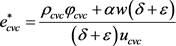${e}_{En}^{\ast }=\frac{{\rho }_{En}{\phi }_{En}+\beta \left(1-w\right)\left(\delta +\epsilon \right)}{\left(\delta +\epsilon \right){u}_{En}}$

4. Stackelberg博弈情形下的最优策略

1、公司创业投资的决策问题

${\prod }_{CVC}\left(t\right)=w{\pi }_{sf}\left(t\right)+{\pi }_{CVCs}\left(t\right)-{C}_{CVC}\left(t\right)$ (7)

$T{\prod }_{CVC}\left({e}_{CVC}\left(t\right)\right)={\int }_{0}^{+\infty }\left(w{\pi }_{sf}\left(t\right)+{\pi }_{CVC}\left(t\right)-{C}_{CVC}\left(t\right)\right){\text{e}}^{-\delta t}\text{d}t$ (8)

$\underset{{e}_{CVC}\left(t\right)}{\mathrm{max}}T{\prod }_{CVC}\left({e}_{CVC}\left(t\right)\right)={\int }_{0}^{+\infty }\left(w{\pi }_{sf}\left(t\right)+{\pi }_{CVCs}\left(t\right)-{C}_{CVC}\left(t\right)\right){\text{e}}^{-\delta t}\text{d}t$ (9)

$\begin{array}{l}\underset{{e}_{CVC}\left(t\right)}{\mathrm{max}}T{\prod }_{CVC}\left({e}_{CVC}\left(t\right)\right)\\ ={\int }_{0}^{+\infty }\left(w\left(\alpha {e}_{CVC}\left(t\right)+\beta {e}_{En}\left(t\right)\right)+{\phi }_{CVC}G-\frac{1}{2}\left(1-\eta \right){u}_{CVC}\left(t\right){e}_{CVC}{\left(t\right)}^{2}\\ \text{\hspace{0.17em}}\text{\hspace{0.17em}}\underset{}{\overset{}{\text{ }}}+{{\prod }^{\prime }}_{CVC}\left(G\right)\left({\rho }_{CVC}{e}_{CVC}\left(t\right)+{\rho }_{En}{e}_{En}\left(t\right)-\epsilon G\right)\right){\text{e}}^{-\delta t}\text{d}t\end{array}$ (26)

2、创业企业的决策

${\prod }_{En}\left(t\right)=\left(1-w\left(t\right)\right){\pi }_{sf}\left(t\right)+{\pi }_{Ens}\left(t\right)-{C}_{En}\left(t\right)$ (10)

$T{\prod }_{En}\left({e}_{En}\left(t\right)\right)={\int }_{0}^{+\infty }\left(\left(1-w\left(t\right)\right){\pi }_{sf}\left(t\right)+{\pi }_{Ens}\left(t\right)-{C}_{En}\left(t\right)\right){\text{e}}^{-\delta t}\text{d}t$ (11)

$\begin{array}{l}\underset{{e}_{En}\left(t\right)}{\mathrm{max}}T{\Pi }_{CVC}\left({e}_{En}\left(t\right)\right)={\int }_{0}^{+\infty }\left(\left(1-w\right)\left(\alpha {e}_{CVC}\left(t\right)+\beta {e}_{En}\left(t\right)\right)+{\phi }_{CVC}G-\frac{1}{2}{u}_{En}\left(t\right){e}_{En}{\left(t\right)}^{2}\\ \text{\hspace{0.17em}}\text{\hspace{0.17em}}\text{\hspace{0.17em}}\text{\hspace{0.17em}}\text{\hspace{0.17em}}\text{\hspace{0.17em}}\text{\hspace{0.17em}}\text{\hspace{0.17em}}\text{\hspace{0.17em}}\text{\hspace{0.17em}}\text{\hspace{0.17em}}\text{\hspace{0.17em}}\text{\hspace{0.17em}}\text{\hspace{0.17em}}\text{\hspace{0.17em}}\text{\hspace{0.17em}}\text{\hspace{0.17em}}\text{\hspace{0.17em}}\text{\hspace{0.17em}}\text{\hspace{0.17em}}\text{\hspace{0.17em}}\text{\hspace{0.17em}}\text{\hspace{0.17em}}\text{\hspace{0.17em}}\text{\hspace{0.17em}}\text{\hspace{0.17em}}\text{\hspace{0.17em}}\text{ }-\frac{1}{2}\eta {u}_{CVC}\left(t\right){e}_{CVC}{\left(t\right)}^{2}+{{\Pi }^{\prime }}_{En}\left(G\right)\left({\rho }_{CVC}{e}_{CVC}\left(t\right)+{\rho }_{En}{e}_{En}\left(t\right)-\epsilon G\right)\right){\text{e}}^{-\delta t}\text{d}t\end{array}$ (27)

${u}_{CVC}\left(t\right)\equiv {u}_{cvc}$${u}_{En}\left(t\right)\equiv {u}_{En}$${\rho }_{CVC}\left(t\right)\equiv {\rho }_{cvc}$${\rho }_{En}\left(t\right)\equiv {\rho }_{En}$${\phi }_{CVC}\left(t\right)\equiv {\phi }_{cvc}$$w\left(t\right)\equiv w$

${e}_{CVC}^{*}=\frac{\alpha \left(2-w\right)\left(\delta +\epsilon \right)+{\rho }_{CVC}\left(2{\phi }_{CVC}+{\phi }_{En}\right)}{2{u}_{CVC}\left(\delta +\epsilon \right)}$

${e}_{En}^{*}=\frac{\left(1-w\right)\left(\delta +\epsilon \right)\beta +{\rho }_{En}{\phi }_{En}}{\left(\delta +\epsilon \right){u}_{En}}$

${\eta }^{\text{*}}=\frac{\alpha \left(2-3w\right)\left(\delta +\epsilon \right)+{\rho }_{CVC}\left(2{\phi }_{En}-{\varphi }_{CVC}\right)}{\alpha \left(2-w\right)\left(\delta +\epsilon \right)+{\rho }_{CVC}\left(2{\phi }_{En}+{\phi }_{CVC}\right)}$(28)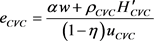(29)

$\begin{array}{l}\delta {H}_{En}=\underset{{e}_{En}\ge 0}{\mathrm{max}}\left\{\left(1-w\right)\left(\alpha {e}_{CVC}\left(t\right)+\beta {e}_{En}\left(t\right)\right)+{\phi }_{En}G-\frac{1}{2}{u}_{En}{e}_{En}{\left(t\right)}^{2}\\ \text{\hspace{0.17em}}\text{\hspace{0.17em}}\text{\hspace{0.17em}}\text{\hspace{0.17em}}\text{\hspace{0.17em}}\text{\hspace{0.17em}}\text{\hspace{0.17em}}\text{\hspace{0.17em}}\text{\hspace{0.17em}}\text{\hspace{0.17em}}\text{\hspace{0.17em}}\text{\hspace{0.17em}}\text{\hspace{0.17em}}\text{\hspace{0.17em}}\text{\hspace{0.17em}}\text{\hspace{0.17em}}\text{\hspace{0.17em}}\text{\hspace{0.17em}}-\frac{1}{2}\eta {u}_{CVC}{e}_{CVC}{\left(t\right)}^{2}+{{H}^{\prime }}_{En}\left(G\right)\left({\rho }_{CVC}{e}_{CVC}\left(t\right)+{\rho }_{En}{e}_{En}\left(t\right)-\epsilon G\right)\right\}\end{array}$ (30)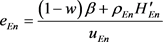(31)

$\eta =\frac{\alpha \left(2-3w\right)+{\rho }_{CVC}\left(2{{H}^{\prime }}_{En}-{{H}^{\prime }}_{CVC}\right)}{\alpha \left(2-w\right)+{\rho }_{CVC}\left(2{{H}^{\prime }}_{En}+{{H}^{\prime }}_{CVC}\right)}$ (32)

${H}_{CVC}\left(G\right)={\lambda }_{1}G+{\lambda }_{2}$ (33)

${H}_{En}\left(G\right)={\varphi }_{1}G+{\varphi }_{2}$ (34)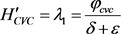(35)

${{H}^{\prime }}_{En}={\varphi }_{1}=\frac{{\phi }_{En}}{\delta +\epsilon }$ (36)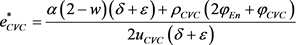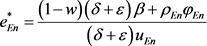${\eta }^{\text{*}}=\frac{\alpha \left(2-3w\right)\left(\delta +\epsilon \right)+{\rho }_{CVC}\left(2{\phi }_{En}-{\phi }_{CVC}\right)}{\alpha \left(2-w\right)\left(\delta +\epsilon \right)+{\rho }_{CVC}\left(2{\phi }_{En}+{\phi }_{CVC}\right)}$

5. 算例分析

Nash博弈下最优CVC创业链声誉函数为${H}_{1}=5G+2350.6$${H}_{2}=10G+1696$

Stackelberg博弈下最优CVC创业链声誉函数为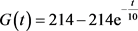${H}_{3}=5G+\frac{81432}{55}$${H}_{4}=10G+\frac{106294}{55}$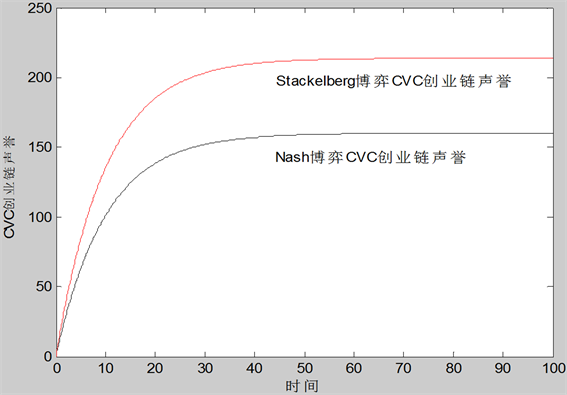Figure 1. Relationship between CVC entrepreneurial chain reputation G(t) and time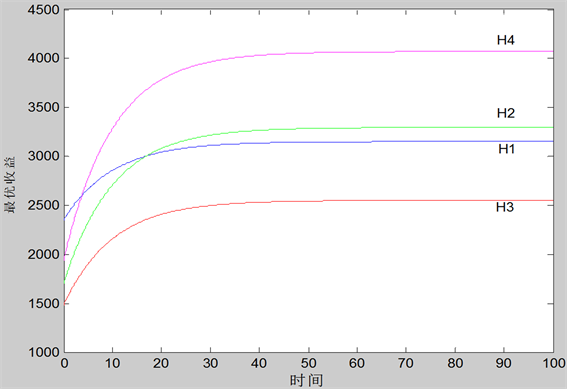Figure 2. Relationship between Time Venture Capital’s and CVC’s Optimal Income and under Two Game Situations

6. 结论

1) 在Nash和Stackelberg博弈均衡条件下，CVC创业链的声誉 $G\left(t\right)$ 与时间皆呈正比的关系，在合作初期，CVC创业链声誉愈来愈好，变化幅度较大，随着合作时间的延长，声誉趋于一个较为稳定的水平。

2) CVC和创业企业的最优收益随着时间的推移而逐渐趋于稳定的状态。在Nash博弈条件下，在合作初期，CVC的最优收益较高，随着合作时间的延长，创业企业获得更多的收益。在Stackelberg博弈条件下，创业企业的最优收益均高于CVC的最优收益。同时，Nash博弈条件下，CVC的最优收益较高，而创业企业的最优收益与Stackelberg博弈条件下的最优收益相比则相对较低。

 王元, 等, 主编. 中创业风险投资发展报告[M]. 北京: 经济管理出版社, 2011.

 Elisa, A.-G. and Gary, D. (2016) Are Entre-preneurial Venture’s Innovation Rates Sensitive to Investor Complementary Assets? Comparing Biotech Ventures Backed by Corporate and Independent VCs. Strategic Management Journal, 37, 819-834.
https://doi.org/10.1002/smj.2359

 Keil (2000) External Corporate Venturing: Speed and Capability Development. Institute of Strategy and International Business, Helsinki University of Technology.

 Kann (2000) Strategic Venture Capital Investing by Corporations: A Framework for Structuring and Valuing Corporate Venture Capital Programs. Dissertation of Stanford University.

 晏钢. 企业战略风险投资研究[D]: [博士学位论文]. 上海: 复旦大学, 2002.

 杨青, 李钰. 风险投资中的双重道德风险与最优合约安排分析[J]. 系统工程, 2004, 131(11): 71-73.

 邓艳红, 陈宏民. 风险投资中的双向道德风险与帕累托有效的合约区间——讨价还价的视角[J]. 经济学, 2006, 5(3): 923-928.

 郑辉. 风险投资中逆向选择的解决——信号传递和信息甄别[J]. 工业技术经济, 2007, 26(3): 131-136.

 Ramy, E. and Arieh, G. (2003) A Multi-Period Game Theoretic Model of Venture Capitalists and Entrepreneurs. Eu-ropean Journal of Operation Research, 144, 440-453.
https://doi.org/10.1016/S0377-2217(02)00144-3

 赵黎明, 刘猛, 郝林娜. 基于创业链声誉的风险投资与风险企业合作的微分对策模型研究[J]. 管理工程学报, 2016, 30(1): 1678-175.

Top